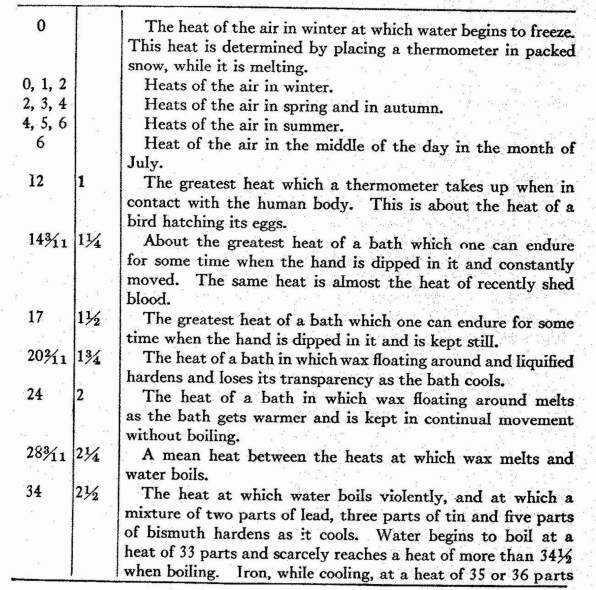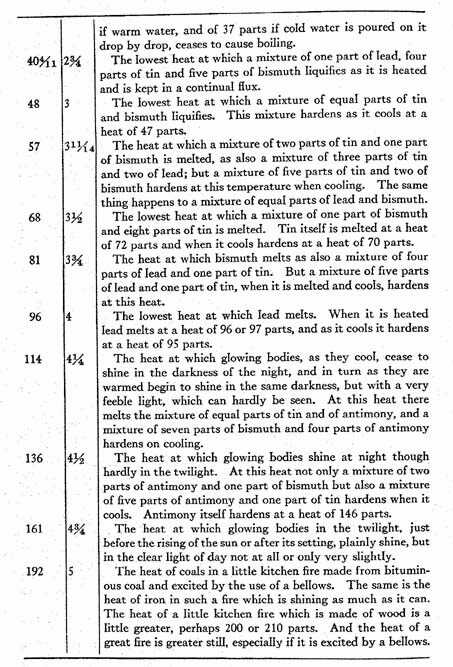A Source Book in Physics

Contents:
Author: Isaac Newton

Show Summary
Physics

# A SCALE OF THE DEGREES OF HeatIn the first column are the degrees of heat in arithmetical progression, computed by beginning with the heat at which water begins to freeze, as from the lowest degree of heat or from the common boundary of heat and cold, and by setting the external heat of the human body at 12 parts. In the second column are the degrees of heat in geometrical progression, so that the second degree is twice as great as the first, the third twice as great as the second, and the fourth twice as great as the third; and the first is the external heat of the human body, which is sensibly constant. It appears by this table that the heat of boiling water is almost three times as great as the heat of the human body, that the heat of melting tin is six times as great, the heat of melting lead eight times as great, the heat of melting antimony twelve times as great and the ordinary heat of a kitchen fire sixteen or seventeen times as great as the heat of the human body.

This table was constructed by the help of a thermometer and of heated iron. With the thermometer I found the measure of all the heats up to that at which lead melts and by the hot iron I found the measure of the other heats. For the heat which the hot iron communicates in a given time to cold bodies which are near it, that is, the heat which the iron loses in a given time, is proportional to the whole heat of the iron. And so, if the times of cooling are taken equal, the heats will be in a geometrical progression and consequently can easily be found with a table of logarithms. First therefore, I found with a thermometer made with linseed oil that if the oil, when the thermometer was placed in melting snow, occupied a space taken as 10000, the same oil expanded by the heat of the first degree, or of the human body, occupied the space 10256, and by the heat of water just beginning to boil the space 10705, and by the heat of water boiling violently the space 10725, and by the heat of melted tin when it begins to harden on cooling and to take on the consistency of an amalgam the space 11516, and when it is completely hardened the space 11496. Therefore the oil was expanded in the ratio of 40 to 39 by the heat of the human body, in the ratio of 15 to 14 by the heat of boiling water, in the ratio of 15 to 13 by the heat of tin which is cooling, when it begins to coagulate and stiffen, and in the ratio of 23 to 20 by the heat at which cooling tin is completely hardened. The expansion of air by an equal heat is ten times as great as the expansion of oil and the expansion of oil is about fifteen steps greater than the expansion of alcohol. And when these were found, by setting the heats proportional to the expansion of the oil and writing 12 parts for the heat of the human body, we obtained for the heat of water when it begins to boil 33 parts, and when it boils more violently 34 parts; and for the heat of tin, either when it melts or when on cooling it begins to stiffen and to take on the consistency of an amalgam, we found 72 parts and when on cooling it hardens completely we found 70 parts. When I had these points determined, in order to investigate the others, I heated a large enough block of iron until it was glowing, and taking it from the fire with a forceps while it was glowing I placed it at once in a cold place where the wind was constantly blowing; and placing on it little pieces of various metals and other liquefiable bodies, I noted the times of cooling until all these bodies lost their fluidity and hardened, and until the heat of the iron became equal to the heat of the human body. Then by assuming that the excess of the heat of the iron and of the hardening bodies above the heat of the atmosphere, found by the thermometer, were in geometrical progression when the times were in arithmetical progression, all the heats were determined. I placed the iron not in quiet air but in a uniformly blowing wind, so that the air warmed by the iron would continually be taken away by the wind, and cold air would come in its place with a uniform motion. For thus equal parts of the air are warmed in equal times and carry away a heat proportional to the heat of the iron. The heats so found had the same ratio to one another as those found by the thermometer and therefore we have correctly assumed that the expansions of the oil were proportional to its heats.

Contents:

### Related Resources

Sir Isaac Newton

Title: A Source Book in Physics

Select an option:

## Email Options

Title: A Source Book in Physics

Select an option: